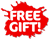Skip to content

# Casio fx-300ES Plus 2nd Edition Scientific Calculator, Blue

by Casio
Condition: New
SKU CASFX300ESPLS2BU
Original price \$13.77 - Original price \$13.77
Original price \$13.77
\$29.99
\$29.99 - \$29.99
Current price \$29.99##### Item Description
• Use Cases: Statistics courses including fraction calculations, statistics, tables
• Natural textbook display: Simplify fractions and roots
• Repeating decimal display: Prime factorization
• Random integer generator: Combinations and permutations

## Overview

The updated fx-300ES Plus 2nd Edition comes with Natural Textbook Display (NTD). NTD displays formulas and results exactly as they appear in the textbook. The fx-300ESPLUS2 also comes in an updated protective hard case. This slim and sleek scientific calculator offers all the functionality needed for middle school mathematics classes and college-level Statistics courses.

### Product Highlights:

Use cases: Statistics courses including fraction calculations, statistics and tables

Natural textbook display: Simplify fractions and roots

Repeating decimal display: Prime factorization

Random integer generator: Combinations and permutations

### Product Features:

Natural Textbook Display

Casio's fx-300ES Plus 2 model can display fractions, derivatives, integrals, sum and other operations similar to their display in math textbooks.

Varied Use Functions

Converts between Standard form and a Decimal, Review/edit previous entries, Random Number Generator.

Table Generation

2-function Table of values [f(x), g(x)]

### Product Specifications:

GENERAL

Power Source - Solar Powered with Battery Back-up

Number of Batteries - 1 LR44 batteries required. (included)

Power Supply - Solar + (G13/LR44)x1

Display (Lines or Dots) - 16 x 4 Lines (96 x 31 Dots)

The updated fx-300ES Plus 2nd Edition comes with Natural Textbook Display (NTD). NTD displays formulas and results exactly as they appear in the textbook. The fx-300ESPLUS2 also comes in an updated protective hard case. This slim and sleek scientific calculator offers all the functionality needed for middle school mathematics classes and college-level Statistics courses.

### Product Highlights:

Use cases: Statistics courses including fraction calculations, statistics and tables

Natural textbook display: Simplify fractions and roots

Repeating decimal display: Prime factorization

Random integer generator: Combinations and permutations

### Product Features:

Natural Textbook Display

Casio's fx-300ES Plus 2 model can display fractions, derivatives, integrals, sum and other operations similar to their display in math textbooks.

Varied Use Functions

Converts between Standard form and a Decimal, Review/edit previous entries, Random Number Generator.

Table Generation

2-function Table of values [f(x), g(x)]

### Product Specifications:

GENERAL

Power Source - Solar Powered with Battery Back-up

Number of Batteries - 1 LR44 batteries required. (included)

Power Supply - Solar + (G13/LR44)x1

Display (Lines or Dots) - 16 x 4 Lines (96 x 31 Dots)

Auto Power OFF - Yes

DISPLAY / EXPRESSION

Natural Textbook Display - Yes

Input - Yes

Output (Fraction) - Yes

Output (pi, root) - Yes

UNDO - No

Transformations Sexagesimal-Decimal

Transformation - Yes

Engineering Notation - Yes

Recurring Decimal - Yes

BASIC FUNCTIONS

Trigonometric, Inverse Trigonometric - Yes

Hyperbolic, Inverse Hyperbolic - Yes

Exponental, Logarithmic (log,ln,10x,ex) - Yes

Power and Radical Root - Yes

Fraction - Yes

Mixed Fraction - Yes

Pi - Yes

Rounding - Yes

GCD/LCM - Yes

Remainder (R÷) - Yes

Base Specified Logarithmic (Log aB) - Yes

Prime Factorization - Yes

Ratio Calculation - No

UTILITIES Variables - 9

Variables List - No

Previous Answer Memory (Pre Ans) - Yes

Engineering Symbol Calculation - No

Solve function - No

CALC Function (Stored Formula Calculation) - No

Scientific Constant - No

Metric Conversion - No

SPECIAL FEATURES

Statistics - Yes

Standard Deviation - Yes

Regression - Yes

Variance - No

Stat Values List - No

Combination, Permutation (nCr, nPr) - Yes

Random Numbers - Yes

Random Integers (RanInt) - Yes

Integer / Nearest Lower Integer (Int,Intg) - Yes

Table Function - Yes

Type of Table - f(x), g(x)

Show Less

## Box Includes

• Casio fx-300ES Plus 2nd Edition Scientific Calculator, Blue
• Authorized Casio Dealer Limited Warranty
x